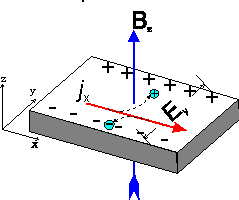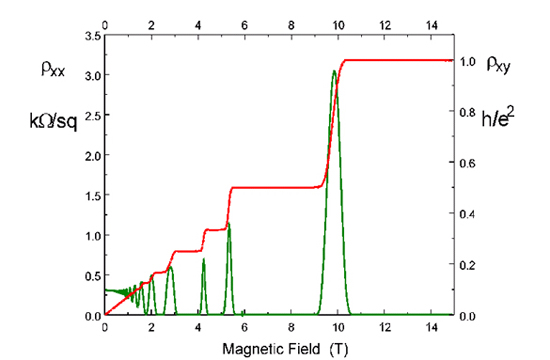## "Classical" electrodynamics is boring because

• it's a dead subject (it hasn't changed much in the last 100 years)
• we've studied it before, we know everything already
• there are much more interesting forces in nature

Galvani (1780s)

Aldini (1800s)

Nijmegen (2000s)

## Energetics of fission reaction: U-235

 kinetic energy of neutrons $$\sim 2$$ MeV gamma ray photons $$\sim 7$$ MeV kinetic energy of nuclei $$\sim 170$$ MeV

Nuclei fly apart at about 3% of light speed
because of Coulomb repulsion

## Important aspects of electric charges and classical electrodynamics

• charge comes in two different varieties (+ and -)
• charge is quantized in discrete units
• charge is conserved (locally)
• force has inverse square form
• forces display linear superposition

## Elementary charge: how accurately is it known?

Theresa Knott, CC BY-SA 3.0, via Wikimedia Commons

Millikan's oil droplet experiment (1909; Nobel 1923):

$$1.5924(17) \times 10^-19$$ C (accurate to 1% of current value)

## Most accurate way of measuring $$e$$

combine the…

### Josephson effect (voltage to frequency)

$$I(t) = I_c \sin \phi(t)$$, $$\frac{d\phi}{dt} = \frac{\hbar}{2e} V(t)$$

and the…

### Quantum Hall effectTogether: $$2 \times \frac{h}{2e} \times \frac{e^2}{h} = e = 1.602176487(40) \times 10^{-19} C$$

## How accurately is charge quantized?

Published experiments: $$\frac{|e_{el}| - e_p|}{|e_{el}|} \lesssim 10^{-21}$$

Proposed experiment using cold atoms, lasers and interferometry: $$\frac{|e_{el}| - e_p|}{|e_{el}|} \lesssim 10^{-28}$$

## How accurate is Coulomb's law?

Possible deviations:

• the force is not $$\frac{1}{r^2}$$ but $$\frac{1}{r^{2 + \epsilon}}$$
• the potential has a Yukawa form, $$\frac{e^{-\mu r}}{r}$$ where $$\mu = m_\gamma c/\hbar$$ ('massive' photon)

## Cavendish's experiment

See the account of Cavendish's extraordinary work, by none other that J. C. Maxwell, in The electrical researches of the Honourable Henry Cavendish

## Most quoted "recent" experiment

Williams, Faller, Hill 1971

$$\epsilon = (2.7 \pm 3.1) \times 10^{-16}$$

Revisited by Fulcher (1986):

$$\epsilon = (1.0 \pm 1.2) \times 10^{-16}$$

## Tests of the photon mass

• terrestrial measurements of $$c$$ at different frequencies
• measurement of radio dispersion in pulsar signals
• lab tests of Coulomb's law
• limits on constant external magnetic field at Earth's surface (Schrödinger!)

## Tests of the photon mass (recent)

Could exist down to a scale given by Heisenberg's uncertainty principle: (age of universe $$\simeq 10^{10}$$ years)

$$m_\gamma \simeq \frac{\hbar}{(\Delta t) c^2} \simeq 10^{-66} g$$

## Is the electron really a point-like particle?

Suppose that it is. Problem: field energy diverges!

$u = \frac{\varepsilon_0}{2} E^2 = \frac{e^2}{32 \pi \varepsilon_0 r^4}$ $\rightarrow U = \int dr \frac{e^2}{8 \varepsilon_0 r^2} \rightarrow \infty$

## Is the electron really a point-like particle?

Suppose it has a radius $$a$$.

Good! Energy is finite.

Moreover: electromagnetism gives inertia (a mass!) to the electron!

## Electromagnetic mass

Suppose that the electron is a shell of negative charge

Accelerated electron displays inertia because of retardation effects of EM self-interaction

Thomson, Heaviside, Lorentz, Poincaré, Fermi, Wilson, etc

When particles come very close to each other:

EM fields become very large!

Maybe the true equations are nonlinear?

Infinite self-energy of point-like electron resolved by nonlinearity?

Famous attempt at classical nonlinear theory of E&M: Born & Infeld (1934)

Actually, quantum electrodynamics provides nonlinear effects

## Summary

There remain many improperly understood things within electrodynamics

Although remarkably wide, the regimes of validity of the laws of electrodynamics might still be limited

## Summary

Discovery of a failure of ED would have very interesting consequences:

• loss of gauge invariance (charge conservation?)
• speed of light frequency-dependent
• special relativity not correct as formulated